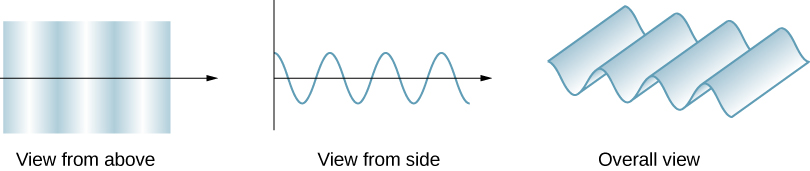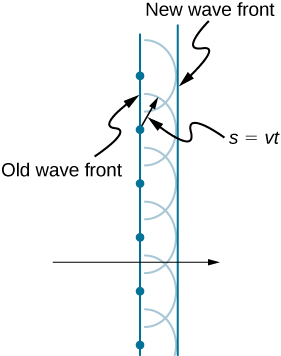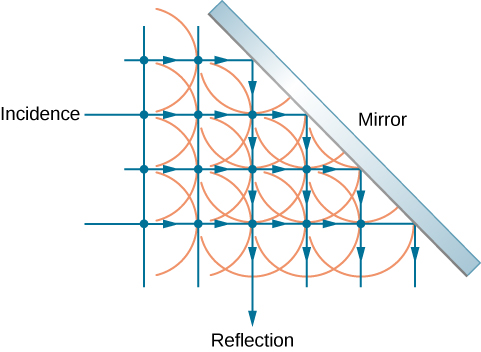# 1.6 Huygens’s principle

 Page 1 / 4
By the end of this section, you will be able to:
• Describe Huygens’s principle
• Use Huygens’s principle to explain the law of reflection
• Use Huygens’s principle to explain the law of refraction
• Use Huygens’s principle to explain diffraction

So far in this chapter, we have been discussing optical phenomena using the ray model of light. However, some phenomena require analysis and explanations based on the wave characteristics of light. This is particularly true when the wavelength is not negligible compared to the dimensions of an optical device, such as a slit in the case of diffraction . Huygens’s principle is an indispensable tool for this analysis.

[link] shows how a transverse wave looks as viewed from above and from the side. A light wave can be imagined to propagate like this, although we do not actually see it wiggling through space. From above, we view the wave fronts (or wave crests) as if we were looking down on ocean waves. The side view would be a graph of the electric or magnetic field. The view from above is perhaps more useful in developing concepts about wave optics    .A transverse wave, such as an electromagnetic light wave, as viewed from above and from the side. The direction of propagation is perpendicular to the wave fronts (or wave crests) and is represented by a ray.

The Dutch scientist Christiaan Huygens (1629–1695) developed a useful technique for determining in detail how and where waves propagate. Starting from some known position, Huygens’s principle    states that every point on a wave front is a source of wavelets that spread out in the forward direction at the same speed as the wave itself. The new wave front is tangent to all of the wavelets.

[link] shows how Huygens’s principle is applied. A wave front is the long edge that moves, for example, with the crest or the trough. Each point on the wave front emits a semicircular wave that moves at the propagation speed v . We can draw these wavelets at a time t later, so that they have moved a distance $s=vt.$ The new wave front is a plane tangent to the wavelets and is where we would expect the wave to be a time t later. Huygens’s principle works for all types of waves, including water waves, sound waves, and light waves. It is useful not only in describing how light waves propagate but also in explaining the laws of reflection and refraction. In addition, we will see that Huygens’s principle tells us how and where light rays interfere.Huygens’s principle applied to a straight wave front. Each point on the wave front emits a semicircular wavelet that moves a distance s = v t . The new wave front is a line tangent to the wavelets.

## Reflection

[link] shows how a mirror reflects an incoming wave at an angle equal to the incident angle, verifying the law of reflection. As the wave front strikes the mirror, wavelets are first emitted from the left part of the mirror and then from the right. The wavelets closer to the left have had time to travel farther, producing a wave front traveling in the direction shown.Huygens’s principle applied to a plane wave front striking a mirror. The wavelets shown were emitted as each point on the wave front struck the mirror. The tangent to these wavelets shows that the new wave front has been reflected at an angle equal to the incident angle. The direction of propagation is perpendicular to the wave front, as shown by the downward-pointing arrows.

Mathematical expression of principle of relativity
given that the velocity v of wave depends on the tension f in the spring, it's length 'I' and it's mass 'm'. derive using dimension the equation of the wave
What is the importance of de-broglie's wavelength?
he related wave to matter
Zahid
at subatomic level wave and matter are associated. this refering to mass energy equivalence
Zahid
how those weight effect a stable motion at equilibrium
how do I differentiate this equation- A sinwt with respect to t
just use the chain rule : let u =wt , the dy/dt = dy/du × du/dt : wA × cos(wt)
Jerry
I see my message got garbled , anyway use the chain rule with u= wt , etc...
Jerry
de broglie wave equation
vy beautiful equation
chandrasekhar
what is electro statics
when you consider systems consisting of fixed charges
Sherly
Diagram of the derive rotational analog equation of v= u+at
what is carat
a unit of weight for precious stones and pearls, now equivalent to 200 milligrams.
LoNE
a science that deals with the composition, structure, and properties of substances and with the transformations that they undergo.
LoNE
what is chemistry
what chemistry ?
Abakar
where are the mcq
ok
Giorgi
acids and bases
Navya
How does unpolarized light have electric vector randomly oriented in all directions.
unpolarized light refers to a wave collection which has an equal distribution of electric field orientations for all directions
pro
In a grating, the angle of diffraction for second order maximum is 30°.When light of wavelength 5*10^-10cm is used. Calculate the number of lines per cm of the grating.
OK I can solve that for you using Bragg's equation 2dsin0over lander
ossy
state the law of gravity 6
what is cathodic protection
its just a technique used for the protection of a metal from corrosion by making it cathode of an electrochemical cell.
akifByBy